## Tuesday, March 29, 2011

### Manifolds: Coordinates

This post is part of a series on Manifolds. Before reading this post, you may refer to the first and second parts of the series for more information.

Another way to define a unique point on a manifold is by the use of coordinate systems. Coordinate systems are collections of numbers (a1, a2... an) that tell one how to identify a point uniquely. There are a number of ways to do this, but within each and every system lies the dimension of the manifold, for the number of coordinates needed to define a point uniquely is the same as the number of dimensions of the manifold.

There are many possible coordinate systems, and some may be more effective on some manifolds than on others. Consider the circle, the simplest of all manifolds.The circular coordinate system (click to enlarge). The angle "a" uniquely determines a point on the circle, and the circle is therefore a one-dimensional manifold. Note that, similar to the difference between the sphere and the ball, the circle is only the line itself, while the interior of the plane defined by the circle is known as a disc. a is taken to be an angle between 0 and 2π if each point on the circle is to have a unique coordinate.A similar process works for the line, an infinite manifold where the (signed) distance from 0 defines a unique point. The above is also called the number line.

For two dimensions, one can combine distance and angle coordinates in several combinations.

For the plane, an infinite two-dimensional manifold, two distance coordinates, taken in perpendicular directions, uniquely define a point (see below). The system, usually using x and y for the directions of coordinates, or axes, is called the Cartesian system. Points are found by following the x-axis for a distance b, and then moving perpendicularly (parallel to the y-axis) some distance c. The point is labeled (b,c).For the aforementioned disc, the angular and linear (distance) systems are combined.The figure above is essentially equivalent to the circular system mentioned previously, with one exception. An additional coordinate, r, is added to denote the distance from the center O of the disc. In reality, the distance r can be arbitrarily large, and the disc therefore infinite, making the angular-linear or polar coordinate system work for the plane as well. However, the restriction of r to being less than or equal to the radius of the disc emphasizes the different construction of the polar system versus the Cartesian system, and how it builds off the circular coordinate system.The final simple possibility is the double angular or spherical system, shown above. It expands off the angular system of the circle, constructing another plane perpendicular to the plane containing the circle. The angle of elevation off the plane serves as the second angle, θ, in addition to the original circular angle, φ.

For three dimensions, there are several more cases.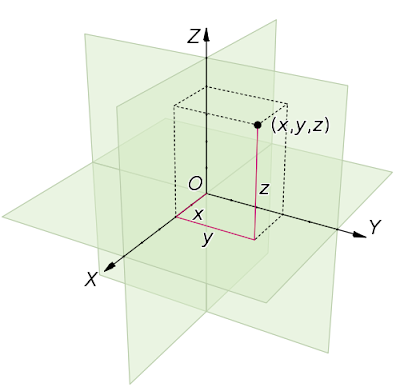The first is the triple linear system, with three axes, x, y, and z. Distances in the direction of each axis are given to uniquely determine a point. (negative coordinates signify movement in the opposite direction)The second possibility is a system with two linear coordinates and one angular coordinate. A manifold using this system is the solid cylinder, or rod. The rod system is based off the disc system, with the angular and first linear coordinates defining a disc, and the second linear coordinate defining distance up the rod. In the above views, the rod is not solid, and instead two circular cross sections are shown to emphasize the first linear dimension. If one was to disregard the first linear dimension, a normal cylinder would result, and this is therefore another possibility for the two dimensional angular system. Consequently, the cylinder is a 2-manifold, and the rod is a 3-manifold. Again, both linear dimensions are restricted to finite values for emphasis.The system with two angular coordinates and one linear coordinate is the ball system, as the ball is the simplest manifold with this system. The ball, as previously mentioned, is simply a solid sphere, with identical angular dimensions. A (restricted) linear dimension is added to denote the distance from the center of the ball, uniquely identifying a point.

The final possibility is the notion of a system with three angular coordinates. Following the pattern of the circle and the sphere, this manifold should also be the set of points equidistant to a fixed point. The set of manifolds with this property are generally called n-spheres, where n is the number of dimensions in the manifold. The circle is then the 1-sphere, the sphere the 2-sphere, and this new manifold, the 3-sphere. However, the full geometry of the three-sphere cannot be expressed with three dimensions, and instead requires four to reveal its curvature.

This generation of manifolds can continue indefinitely through any dimension, but the above process only produces a limited number of manifolds, i.e. the simplest of every dimension. Also, there are multiple possibilities for each system, with the disc and cylinder being one example given above. There are many other similar pairs in higher dimensions when one changes the orientation of the linear and angular dimensions.

Coordinates are necessary in performing mathematical operations on manifolds, and in mapping between them. The differences between angular and linear coordinates, and the number of dimensions needed to express them open the gates to a limitless world of surfaces, which the above method has only begun to explore. For the next post, see here.

Sources: http://www.ibiblio.org/links/devmodules/shared/html/glossary.html, http://www.mathematic.ws/, http://en.wikipedia.org/wiki/Spherical_coordinate_system, Visual Complex Analysis by Tristan Needham,

## Monday, March 21, 2011

### Manifolds: Mappings and Projection

To understand some terms used in this post, it is recommended that you first read Manifolds: Geometrically Equivalent vs. Topologically Equivalent.

The world of manifolds is extremely rich and diverse, especially when higher dimensions are considered. A multitude of objects can be explored, most of them fundamentally different from one another, and we are still very far away from identifying all of them, even in relatively low dimensions.

Note: Before exploring manifolds, it is useful to define "dimension" and what it means in terms of a manifold. Recall the definition of a manifold: any surface that appears "flat" at sufficiently small scale. However, "flat" is another word for Euclidean (or plane) geometry, and we can then define the dimension of a manifold as follows:

If a manifold resembles n-dimensional Euclidean space at sufficiently small scale, then it is an n-dimensional manifold.

As an example of this, consider a typical sphere, such as the Earth (the Earth is not exactly spherical, but is often used to represent the abstract mathematical sphere). To someone standing on the Earth, its surface appears to be a flat plane, i.e. two dimensional Euclidean geometry! Therefore, we conclude that the sphere is a two dimensional manifold, or 2-manifold.

Note: The term "sphere" only includes the surface of the sphere (or the Earth) and does not include the inside of the sphere. The region of three dimensional space bounded by the two dimensional sphere is known as the ball, and is a 3-manifold.

With the above clarification of terms, one notices that is easy to define the Earth as a 2-manifold. However, when one attempts to map the surface of the Earth on a flat surface, there are inevitable distortions.

As an example, consider the Mercator projection of the Earth.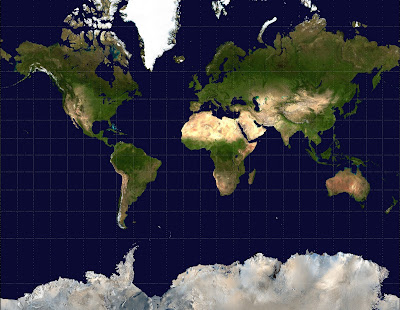When mapping the Earth onto a flat Euclidean plane, one must consider various geometrical properties of the sphere, including area, latitude and longitude lines, and lengths of these lines. A process called projection allows one to view a surface on a flat two dimensional plane. In the above case, a cylinder is used to project the Earth onto a plane (see image below).For each point on the sphere, a line is drawn from the center of the sphere outward through the aforementioned point, and this line will eventually intersect with the cylinder (the cylinder does not actually have a "top" or a "bottom" but rather goes on forever). After all the points are mapped, the cylinder is unrolled into a plane, resulting in the fact that going off the right edge of the map goes to the left edge and vice versa. However, when one chooses a point near one of the poles, the line must go a large distance before intersecting the cylinder, and the poles themselves cannot be mapped at all! Despite these problems, this mapping is desirable for bearings, as it maps rhumb lines (or lines bearing in a specific direction, e.g. northwest, east southeast, etc.) and therefore also preserves angles.

Another (perhaps even simpler) useful projection for mapping the Earth (and especially mathematics) is the stereographic projection. It again maps the sphere onto a flat Euclidean plane, but in a different way. The figure below denotes this.To project stereographically, a point of projection is first chosen. For the purposes of this example, the point of projection is always assumed to be the north pole. For each point on the sphere, a line is drawn from the north pole through this point, and is extended until it intersects the plane passing through the equator of the sphere. In mathematics, this is taken to be the complex plane (see here for a basic discussion of i and the complex plane) and the circle at which the sphere intersects the plane is taken to be the unit circle, i.e. the circle centered at 0 with radius 1.

The figure above shows two arbitrary points. Point A, on the plane outside the unit circle, corresponds to a point on the upper half of the sphere, while Point B, on the plane inside the unit circle, corresponds to a point on the lower half of the sphere. Points on the unit circle obviously remain in the same position. (1 and i are shown as examples)

Additional properties include the south pole of the sphere corresponds to the origin of the plane, and the north pole of the sphere does not correspond to ANY point on the normal plane. This is because the point of projection (the north pole) and the point to be projected (also the north pole) coincide, and the line is therefore a tangent line, which is parallel to the plane and never intersects it. The north pole is sometimes called infinity for this reason.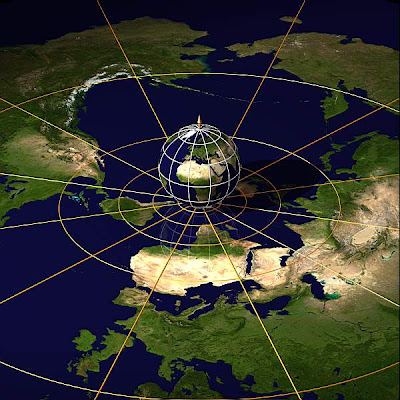An image of the Earth using another type of stereographic projection where the plane of projection is tangent to the south pole of the sphere. In all other respects, this projection is similar to the one above; it still matches every point on the sphere with one point on the plane, with the exception of the north pole.

The stereographic projection has many properties that make it valuable to mathematics, the most important of which is that it preserves circles and angles (for a proof, see sources, specifically Needham). And since the points are projected continuously with a one-to-one correspondence, the mapping is a homeomorphism. Therefore, we can make the statement that

The Euclidean two dimensional plane is homeomorphic to the two dimensional sphere with one point removed.

The above reflects that the point of projection itself (the north pole in the above examples) cannot be projected onto the plane. In mathematics, it is possible to remedy this, by including infinity itself as a point on the complex plane, and the new entity that results is known as the extended complex plane. Infinity then corresponds to the north pole under stereographic projection. Note that the actual direction, in which we approach infinity does not matter, as all lines heading away from the origin will travel upwards on the sphere and eventually reach the north pole. In a sense, infinity is an endpoint of any straight line in the extended complex plane.

A generalized view on stereographic projection allows one to project from spheres of any dimension to their corresponding planes. For example, elliptic polychora, or four dimensional finite polytopes (see the polytopes series) are actually tilings of the three dimensional sphere which is the surface enclosing the four dimensional ball (this is the higher dimensional analog of the two dimensional sphere being a surface enclosing a three dimensional ball). Therefore, stereographic projection can be used in a similar way as the above to project such polychora onto flat Euclidean three space.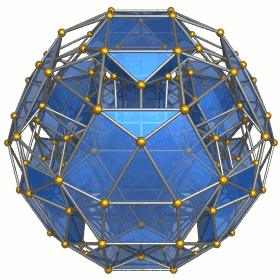An example of a convex polychoron projected stereographically (specifically, it is the cantellated 24-cell, see here for more information)

Mappings and projection have revealed that the sphere and plane are fundamentally different, despite being members of the same dimension. Further posts in the manifolds series addresses this topic (see next post).

Sources: http://en.wikipedia.org/wiki/Stereographic_projection, Visual Complex Analysis by Tristan Needham, http://en.wikipedia.org/wiki/Mercator_projection

## Wednesday, March 9, 2011

### Manifolds: Geometrically Equivalent vs. Topologically Equivalent

A manifold is the general term for a geometric figure, surface, or space. Manifolds can be of any dimension, and of are great importance in mapping, and in mathematics.

The study of the manipulation of surfaces is known as topology. It is very important to understand that geometry and topology, although both dealing with geometric figures, are very different. Geometry is concerned with sizes and shapes, i.e. measuring area, radius, perimeter, volume, and so forth. However, topology, which is more relevant to this post, does not worry about specific shapes. The following definitions are very important distinctions.

Geometrically equivalent: Two objects are geometrically equivalent if they have exactly the same size, shape and dimension. Figures with this property are called congruent. Another identical statement is that if two figures can be placed on top of each other to exactly line them up, then they are congruent.

Homotopic: Two objects are homotopic if they can be continuously deformed into one another. This means that the stretching, bending or twisting of an object does not alter it topologically. Two objects that can be continuous deformed into each other in this way are called homotopic to one another. Therefore, there exists what is called an invariant in the original surface. An invariant is defined in this sense as a feature of a manifold that remains the same when it is changed is some way. The specific example of this for two homotopic manifolds is called a homotopy group.

There are actually several different homotopy groups, each of which defines features of a manifold. The simplest of these is called the fundamental group, which determines the number of holes in a surface. The mug and the torus below both have one hole in their surface; they are homotopic and equivalent topologically.Another way to express the same idea is to consider a point on a manifold, and, starting from that point, trace any path on the given manifold, with one condition: the endpoint and the starting point of the path must coincide. When this happens, the path is called a loop. If all possible loops can be contracted into a point without leaving the given surface, than the surface has no holes and is what is called simply connected.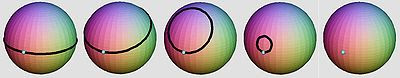A demonstration that the sphere is simply connected. The loop shown, along with any other loop beginning from any other point on the sphere, can be contracted without leaving the surface.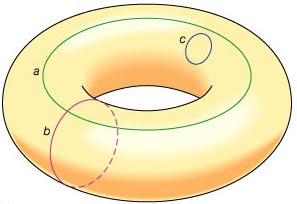An image of a regular torus, with three example loops drawn on its surface. It can easily be seen that some loops, such as c can be contracted to a point without leaving the surface. Others, such as a and b, cannot. Additional analysis of the set of loops on a surface yields the number of holes, known as the genus of a surface.The triple torus is a manifold with genus 3.

The remaining homotopy groups are, simply put, higher dimensional generalizations of this. For example, the 2nd homotopy group deals with the cutting of spheres out of a surface, unlike the fundamental or first group, which deals with the cutting of circles. Therefore, there are infinitely many homotopy groups possible, each of which corresponding to a specific dimension. The "loops" for the 2nd homotopy groups will be surfaces, rather than lines, that can be contracted to a point. In addition, there is a equivalent for any dimension. For any specific manifold, the homotopy groups will assign an invariant (or a set of invariants if multiple groups are used) that identifies it as homotopic to any other manifold with an equivalent invariant.

Homeomorphic: However, the actual definition of topologically equivalent is even broader than this. Two manifolds are topologically equivalent if they are homeomorphic. Note that if two manifolds are homeomorphic, they are homotopic, but the reverse is not necessarily true.

Two manifolds are homeomorphic if there exists some function that maps each point on one manifold to a corresponding point on the other.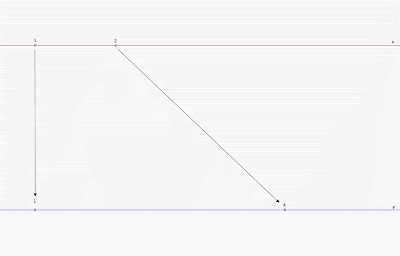An example of a simple mapping (click the image to enlarge) of the number line x (red) to the number line y=x^2 (blue). Each point on x is mapped to a corresponding point on y (only the points x=1 and x=2, becoming y=1 and y=4, respectively, are shown). The above lines are actually manifolds related by the mapping y=x^2!

However, for two manifolds related by a mapping to be homeomorphic, the mapping must satisfy certain conditions, listed below. For the mapping function f that relates a set of points X to a corresponding set of points Y:

1. The function f must be continuous.
2. The function f must be one-to-one.
3. The function f must be onto.
4. The inverse of f must be continuous.

Some definitions are in order:

Continuous: Literally that each change in input causes only a small change in output. In other words, there cannot be any "jumps" or discontinuities in the function.
One-to-one: If the function f maps a point a in X to a specific point b in Y, then a is the only value in X that f maps to b.
Onto: Each value in Y corresponds to a point in X.
Inverse: The inverse of a function f is the mapping that undoes f. In other words, f maps X to Y, and the inverse of f maps Y to X.

Armed with the ideas of homotopic, homeomorphic, and mapping, one can begin to explore the world of manifolds. (see the next post)

Sources: http://en.wikipedia.org/wiki/Homotopy_groups and other various wikipedia titles, Visual Complex Analysis by Tristan Needham, The Poincare Conjecture by Donal O'Shea, http://www.regentsprep.org/Regents/math/algtrig/ATP5/OntoFunctions.htm, http://media.web.britannica.com/eb-media/58/96258-004-7747AF96.jpg

## Saturday, March 5, 2011

### Gamma Rays

Gamma rays are the final type of radiation found on the electromagnetic spectrum. Gamma rays have the highest frequency and smallest wavelength of any radiation, and therefore also have the highest energy.

Gamma rays are powerful enough to penetrate most substances, and are powerful ionizers. The gamma ray spectrum involves wavelengths lower than one trillionth of a meter (less than .000000000001 meters).

This radiation is often produced as a byproduct of atomic decay, in which unstable atoms emit energy through gamma rays and other particles before settling to a stable state. Descriptions of some of these reactions can be found here.

Among the parts of the electromagnetic spectrum, gamma rays are comparatively rare. Few objects are powerful enough to give off high amounts of gamma ray energy. However, this radiation is very important on atomic scales, when atomic decay spontaneously converts mass into energetic photons, i.e. gamma rays. This is possible due to the well known mass-energy relation

E=mc^2

where E corresponds to energy, m to mass, and c a constant (the speed of light: 186282 miles per second). The meaning of this equation is that a certain amount of mass is equal to a certain amount of energy. During the early stages of the Universe, the temperature was sufficiently high that particles collided with their antiparticle counterparts. When they collide, they instantly annihilate each other, releasing energy. As energy, the reverse happened, with particles and antiparticles being spontaneously created. Now these reactions only take place in extreme conditions, such as on the edges of a black hole.

Despite this, astronomical gamma ray sources can still be found, and the closest is the Moon.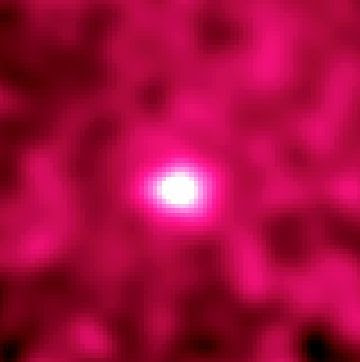An image of the Moon taken by a gamma ray telescope. The gamma rays emitted from the Moon originate when other solar ionizing radiation, such as ultraviolet, hits the Moon and causes the excitation of heavy atoms, and this in turn, causes the emission of gamma rays. In contrast, the Sun is nearly invisible in the gamma ray spectrum, because it consists of very light atoms (mostly Hydrogen and Helium) that cannot be excited by ionizing radiation.

Other gamma ray sources include solar flares, the death of massive stars (through processes such as supernovae) and their remnants (neutron stars and black holes), as well as active galaxies. Galaxies are "active" if large amounts of infalling matter are feeding their central black hole, giving off enormous amounts of radiation. Most information on gamma ray bursts is theoretical, as little is actually known about their sources, and they usually are very distant.

Gamma ray bursts and their sources are some of the most fascinating areas of astronomy.

Sources: http://en.wikipedia.org/wiki/Gamma_ray, http://science.hq.nasa.gov/kids/imagers/ems/gamma.html, http://en.wikipedia.org/wiki/Timeline_of_the_Big_Bang, http://en.wikipedia.org/wiki/Mass–energy_equivalence